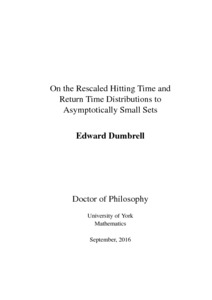# On the Rescaled Hitting Time and Return Time Distributions to Asymptotically Small Sets

Dumbrell, Edward Mark (2018) On the Rescaled Hitting Time and Return Time Distributions to Asymptotically Small Sets. PhD thesis, University of York.Preview
Text
20181112 EDThesis FINAL SUBMITTED 2.pdf - Examined Thesis (PDF)
Consider a hyperbolic flow $\phi_t:M\to M$ on a smooth manifold $M$, and a sequence of open balls $(\D_n)_{n\in\N}$ with $\D_n \subset M$ and measure $m(\D_n) >0$ but also satisfying $\lim_{n \to \infty}m(\D_n)=0$. The expected time it takes for the flow to hit the set $\D_n$, known as the hitting time, or the return time if the flow started in $\D_n$, and each subsequent hit thereafter, is proportional to the measure $m(\D_n)$ of that set, provided the measure is ergodic. In this thesis I study how the distribution of hitting times (and return times), rescaled by an appropriate sequence of constants, converges in the limit. I show conditions under which a Poisson limit law holds by considering the hitting time distributions of an associated discrete dynamical system.